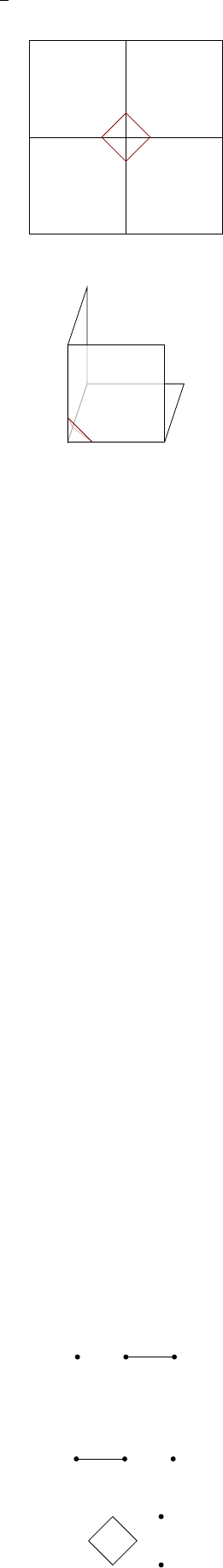5CAT(0) spaces and groups

IV Topics in Geometric Group Theory5.7 Cube complexes
Definition
(Cube complex)
.
A Euclidean complex is a cube complex if every
cell is isometric to a cube (of any dimension).
This is less general than Euclidean complexes, but we can still make high
dimension things out of this. Of course, general Euclidean complexes also work in
high dimensions, but except in two dimensions, the link condition is rather tricky
to check. It turns out the link condition is easy to check for cube complexes.
Purely topologically, the link of each vertex is made out of simplices, and,
subdividing if necessary, they are simplicial complexes. The metric is such that
every edge has length
π
2
, and this is called the “all-right simplicial complex”.
Recall that
is non-positively curved, while
is not.
Definition
(Flag simplicial complex)
.
A simplicial complex
X
is flag if for all
n 2, each copy of
n
in X is in fact the boundary of a copy of
n
in X.
Note that topologically, flag complexes are not special in any sense. For any
simplicial complex K, the first barycentric subdivision is flag.
Theorem (Gromov). A cube complex is non-positively curved iff every link is
flag.
Now the property of being flag is purely combinatorial, and easy to check.
So this lets us work with cube complexes.
Right-angled Artin groups
Definition
(right-angled Artin group)
.
Let
N
be a simplicial graph, i.e. a graph
where the vertices determine the edges, i.e. a graph as a graph theorist would
consider. Then
A
N
= hV (N) | [u, v] = 1 for all (u, v) E(N)i
is the right-angled Artin group, or graph group of N .
Example. If N is the discrete graph on n vertices, then A
N
= F
n
.
Example. If N is the complete graph on n vertices, then A
N
= Z
n
.
Example.
If
N
is a square, i.e. the complete bipartite graph
K
2,2
, then
A
N
=
F
2
× F
2
.
Example.
When
N
is the path with 4 vertices, then this is a complicated group
that doesn’t have a good, alternative description. This is quite an interesting
group.
Definition
(Salvetti complex)
.
Given a simplicial group
N
, the Salvetti complex
S
N
is the cube complex defined as follows:
Set S
(2)
N
is the presentation complex for A
N
.
For any immersion of the 2-skeleton of a
d
-dimensional cube, we glue in
an d-dimensional cube to S
(2)
N
.
Alternatively, we have a natural inclusion
S
(2)
N
(
S
1
)
|V (N)|
, and
S
N
is the
largest subcomplex whose 2-skeleton coincides with S
(2)
N
.
Example. If N is
then A
n
= Z Z
2
, and S
N
is a circle glued to a torus.
Example. If N is
,
then S
N
is T
2
S
1
. There is a unique vertex v, and its link looks like
In fact, there is a recipe for getting the link out of N.
Definition
(Double)
.
The double
D
(
K
) of a simplicial complex
K
is defined as
follows:
The vertices are
{v
+
1
, . . . , v
+
n
, v
1
, . . . , v
n
}
, where
{v
1
, . . . , v
n
}
are the ver-
tices of k.
The simplices are those of the form
hv
±
i
0
, . . . , v
±
i
k
i
, where
hv
i
0
, . . . , v
i
k
i K
.
Example. In the example above, the double of N is just Lk(v)!
Definition
(Flag complex)
.
The flag complex of
N
, written
¯
N
, is the only flag
simplicial complex with 1-skeleton N.
Lemma.
For any (simplicial) graph
N
, the link of the unique vertex of
S
N
is
D(
¯
N). In particular, S
N
is non-positively curved.
Thus, right-angled Artin groups and their Salvetti complexes give examples
of non-positively curved spaces with very general links. It turns out
Theorem.
Right-angled Artin groups embed into
GL
n
Z
(where
n
depends on
N).